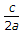# Electronics and Communication Engineering - Microwave Communication

### Exercise :: Microwave Communication - Section 5

11.

In a magnetron the magnetic flux density is of the order of

 A. 1 mWb/s B. 1 Wb/m2 C. 106 m/s D. 1012 m/s

Answer: Option A

Explanation:

No answer description available for this question. Let us discuss.

12.

For a directional coupler the power is in the ratio of

 A. 40 dB B. 30 dB C. 20 dB D. 10 dB

Answer: Option C

Explanation:

No answer description available for this question. Let us discuss.

13.

As regards rectangular and circular waveguides

 A. both have infinite set of TE and TM modes B. rectangular waveguide has infinite TE and TM modes but not the circular waveguide C. circular waveguide has infinite TE and TM modes but not the rectangular waveguide D. none of the above

Answer: Option A

Explanation:

No answer description available for this question. Let us discuss.

14.

In a high frequency coaxial line and the length of line characteristic impedance is a function of

 A. properties of insulating material only B. inner and outer conductor radius only C. both (a) and (b) D. neither (a) nor (b)

Answer: Option C

Explanation:

No answer description available for this question. Let us discuss.

15.

Assertion (A): In TE10 mode the cutoff frequency of rectangular waveguide iswhere a is the broad dimension and c is velocity of light.

Reason (R): TE10 mode is the dominant mode in rectangular waveguide.

 A. Both A and R are correct and R is correct explanation of A B. Both A and R are correct but R is not correct explanation of A C. A is correct but R is wrong D. A is wrong but R is correct

Answer: Option B

Explanation:

No answer description available for this question. Let us discuss.

#### Current Affairs 2021

Interview Questions and Answers